6401.0.60.002 - Information Paper: Increasing the Frequency of CPI Expenditure Class Weight Updates, July 2016
ARCHIVED ISSUE Released at 11:30 AM (CANBERRA TIME) 11/07/2016  First Issue
Page tools:Print All

APPENDIX 2 DERIVING CAPITAL CITY EXPENDITURE WEIGHTS

1. Geographical coverage is a key difference between HFCE (national) and the CPI (metropolitan). As mentioned in paragraph 2.12, the ABS is conducting further work to remove non-metropolitan expenditure to align with the CPI. For the purposes of the example shown below, National HFCE data is used to demonstrate how to utilise data for CPI weighting at the capital city level which would assume metropolitan household expenditure changes are the same as National household expenditure changes.

2. It is proposed to update capital city expenditure weights across the 87 ECs when the most recent HES data becomes available (every six years). The sum of the capital city expenditure aggregates would represent the weighted eight capitals for each EC - this is equivalent to current CPI six yearly re-weighting processes. For the intervening periods between HES years, the expenditure aggregate at the weighted eight capitals level will be updated via the annual movement in expenditure derived from National HFCE data for each EC. This estimate of weighted eight capitals expenditure will then be distributed across each capital city (for every EC) using price updated expenditure aggregates from the previous four quarters.

3.To illustrate the application, an example is included below with reference to September quarter 2011 and September quarter 2012. Table A2.1 shows hypothetical expenditure aggregates of a simplified economy (i.e. three products and cities) following the HES. The capital city expenditure aggregates are then aggregated to derive a new set of expenditure aggregates used for the total of three capitals.

 A2.1 September 2011 link period expenditure aggregates (millions)Sydney Melbourne Brisbane Total (three capitals) Milk EC 40.00 35.00 25.00 100.00 Bread EC 60.00 30.00 30.00 120.00 Vegetables EC 30.00 30.00 30.00 90.00 Total 130.00 95.00 85.00 310.00

4.The expenditure aggregates in A2.1 are used in the compilation of the CPI using the Lowe index formula. In September 2012, suppose prices for each capital city have moved dynamically over the past twelve months which produced the following four quarter average of expenditure aggregates in Table A2.2.

 A2.2 Average expenditure aggregates for December 2011 to September 2012 (millions)Sydney Melbourne Brisbane Total (three capitals) Milk EC 48.00 36.75 25.50 110.25 Bread EC 66.00 31.50 31.20 128.70 Vegetables EC 32.10 30.00 30.00 92.10 Total 146.10 98.25 86.70 331.05

5. National HFCE data is available that indicates the following changes in expenditure have occurred between September 2011 and September 2012: milk (+3.0%), bread (-5.0%) and vegetables (+7.0%). These estimates are used to derive new link period expenditure aggregates for each EC at the weighted three capitals level as shown in Table A2.3.

 A2.3 Weighted three capital city link period expenditure aggregatesSeptember 2011 September 2012 Milk EC 100.00 103.00 Bread EC 120.00 114.00 Vegetables EC 90.00 96.30 Total 310.00 313.30

6. Once new link period expenditure aggregates are derived at the weighted three capital city level, they are distributed across each EC in a manner that preserves each capital city's contribution to the weighted three capital aggregate for the preceding four quarters. The new link period value aggregates for September 2012 are shown below in Table A2.4. For example, the value of \$44.84 million for milk in Sydney is derived by multiplying the proportional expenditure for Sydney in Table A2.2 (48/110.25) by the new link period expenditure aggregate for milk (\$103 million). The expenditure aggregates in Table A2.3 form link period expenditure aggregates from which future period-to-period price change can be derived.

 A2.4 September 2012 link period expenditure aggregates (millions)Sydney Melbourne Brisbane Total (three capitals) Milk EC 44.84 34.33 23.82 103.00 Bread EC 58.46 27.90 27.64 114.00 Vegetables EC 33.56 31.37 31.37 96.30 Total 136.87 93.60 82.83 313.30

7. This approach can be generalised and denoted as follows;

Step 1: Derive weighted eight capital expenditure aggregates using movements in National HFCE.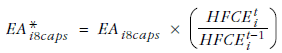Step 2: Pro-rate weighted eight capital expenditure aggregates across capital cities.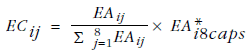Where: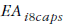= previous weighted eight capitals link period expenditure aggregates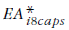= new weighted eight capitals link period expenditure aggregates adjusted using HFCE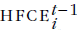= HFCE for the previous year for EC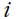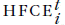= HFCE for the current year for EC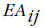= four quarter (DQ,MQ,JQ SQ) average of ECand capital city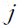updated expenditure aggregate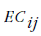= link period expenditure aggregate for ECand capital city.